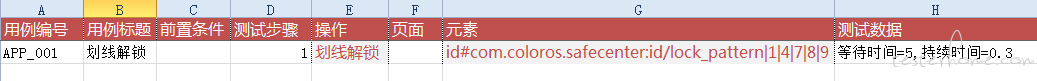# Appium Sweetest 自动化测试：App 划线解锁新姿势

tonglei · July 10, 2018 · Last by xifan89cc replied at July 18, 2019 · 2666 hits

## 引子

Sweetest 开源以来，得到不少同学的喜爱，也有很多同学期望尽快支持 App 测试。

Github 项目地址：https://github.com/tonglei100/sweetest

QQ 交流群：158755338 (验证码：python)

## 需求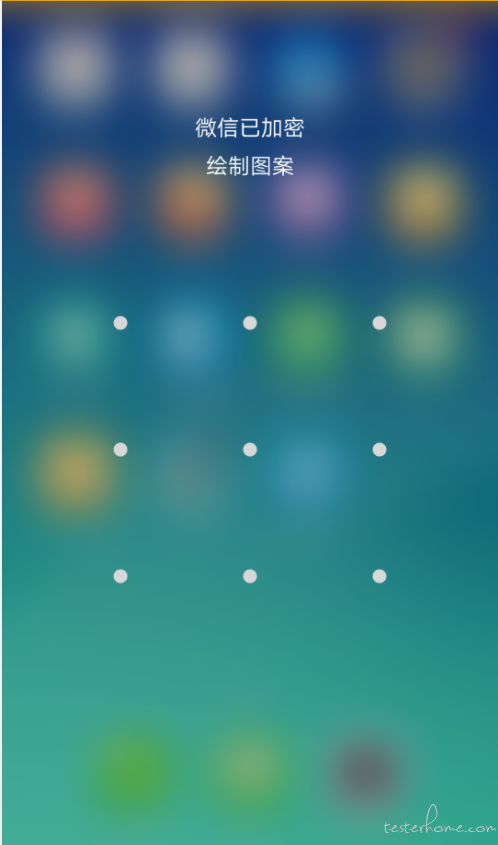## 实现方式

### 一、划线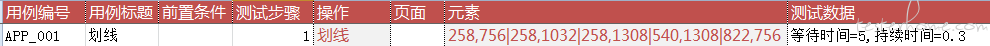### 二、单独实现“划线解锁”操作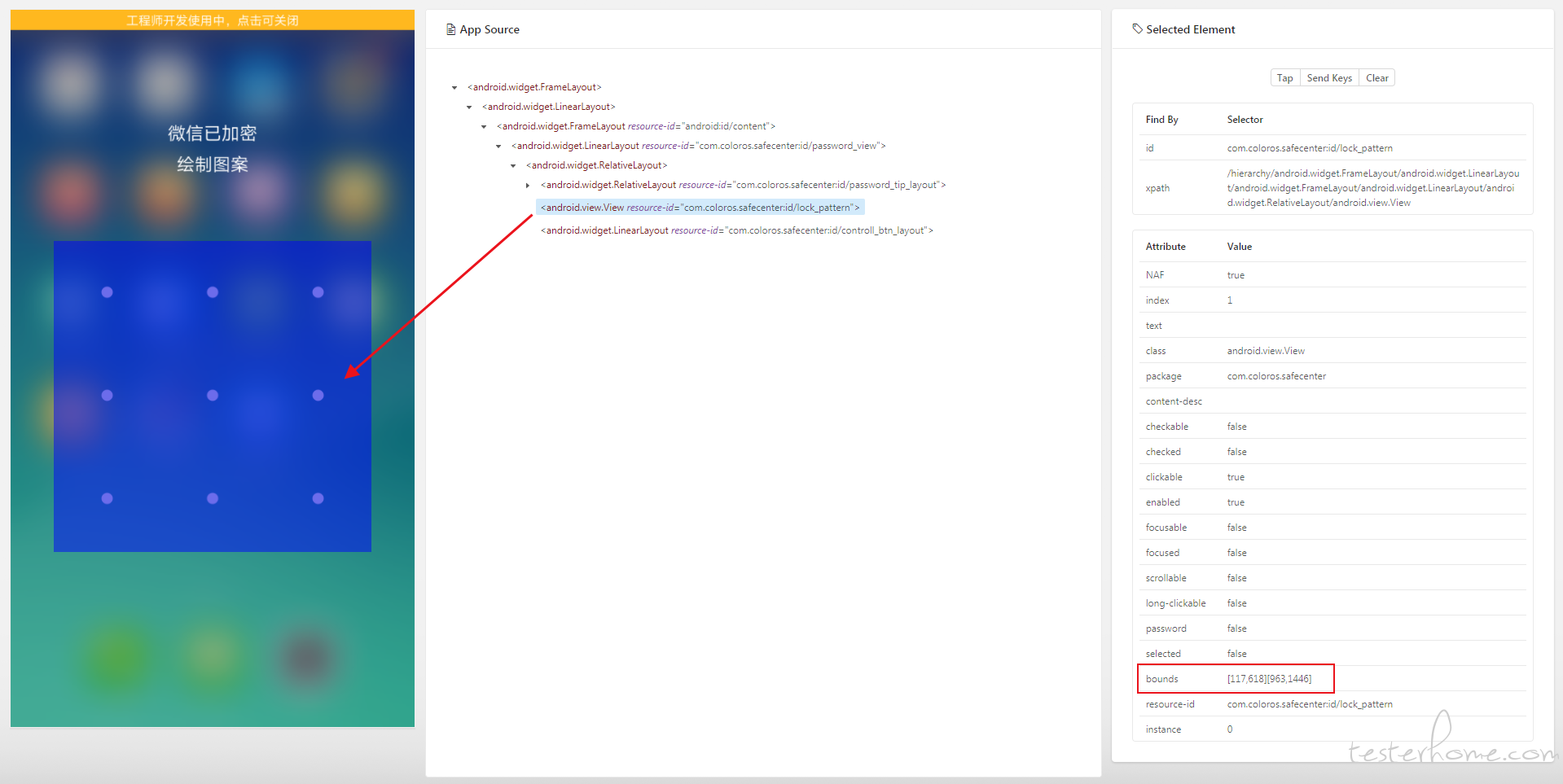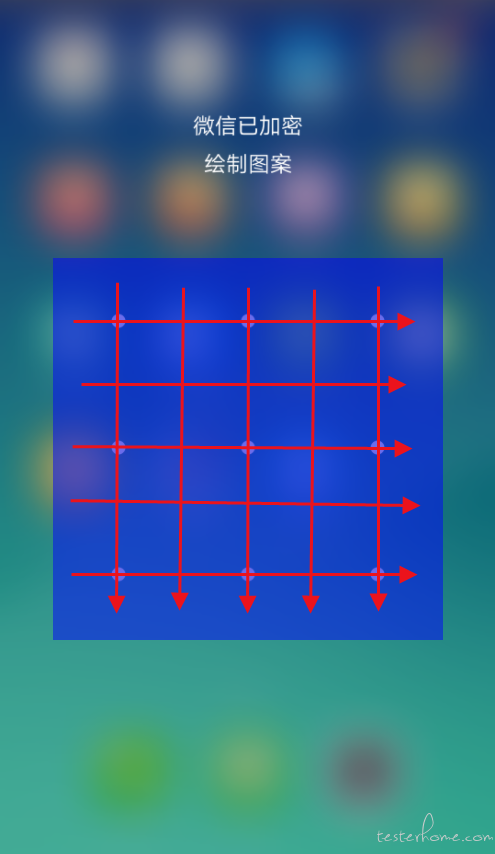#### 2. 用例设计分析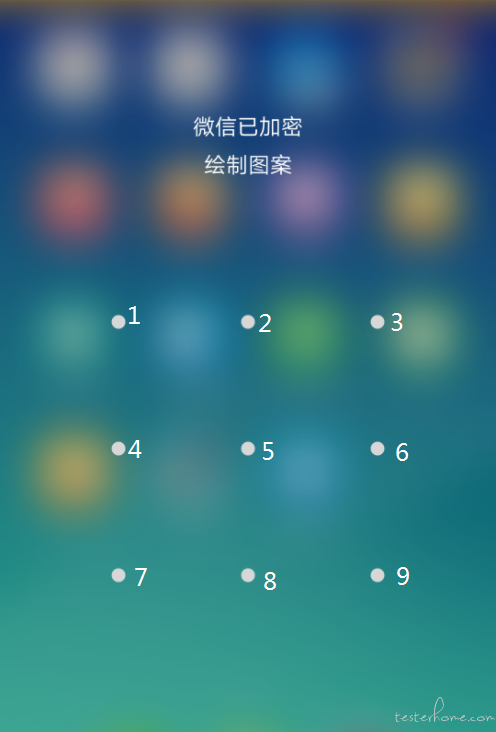``def line_unlock(step):    # 从测试用例中获取元素的定义    element = step['element']    # 每2个点划线的持续时间    duration = float(step['data'].get('持续时间', 0.3))    # 断言    assert isinstance(element, list) and len(element) > 2, '坐标格式或数量不对，正确格式如：lock_pattern|1|4|7|8|9'    # 元素定位    _e = locating_element(element)    # 获取元素的坐标数据    rect = _e.rect    w = rect['width']/6    h = rect['height']/6    # 点的坐标定义    key = {}    key['1'] = (rect['x'] + 1*w, rect['y'] + 1*h)    key['2'] = (rect['x'] + 3*w, rect['y'] + 1*h)    key['3'] = (rect['x'] + 5*w, rect['y'] + 1*h)    key['4'] = (rect['x'] + 1*w, rect['y'] + 3*h)    key['5'] = (rect['x'] + 3*w, rect['y'] + 3*h)    key['6'] = (rect['x'] + 5*w, rect['y'] + 3*h)    key['7'] = (rect['x'] + 1*w, rect['y'] + 5*h)    key['8'] = (rect['x'] + 3*w, rect['y'] + 5*h)    key['9'] = (rect['x'] + 5*w, rect['y'] + 5*h)    # 划线    action = TouchAction(g.driver)    for i in range(1, len(element)):        k = element[i]        if i == 1:            action = action.press(x=key[k], y=key[k]).wait(duration*1000)        action.move_to(x=key[k], y=key[k]).wait(duration*1000)    action.release().perform()``

OK，测试用例如下：